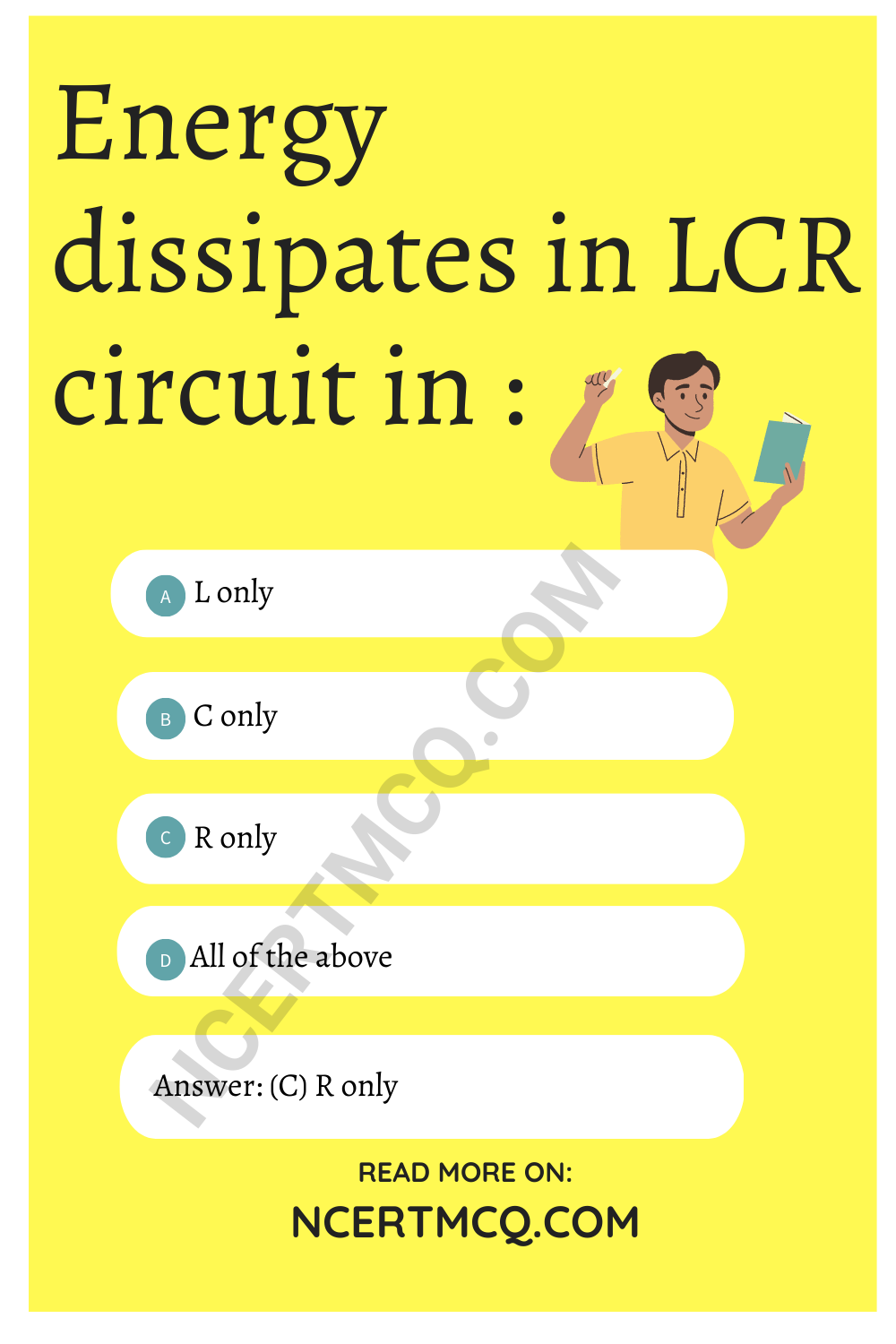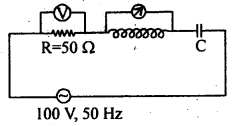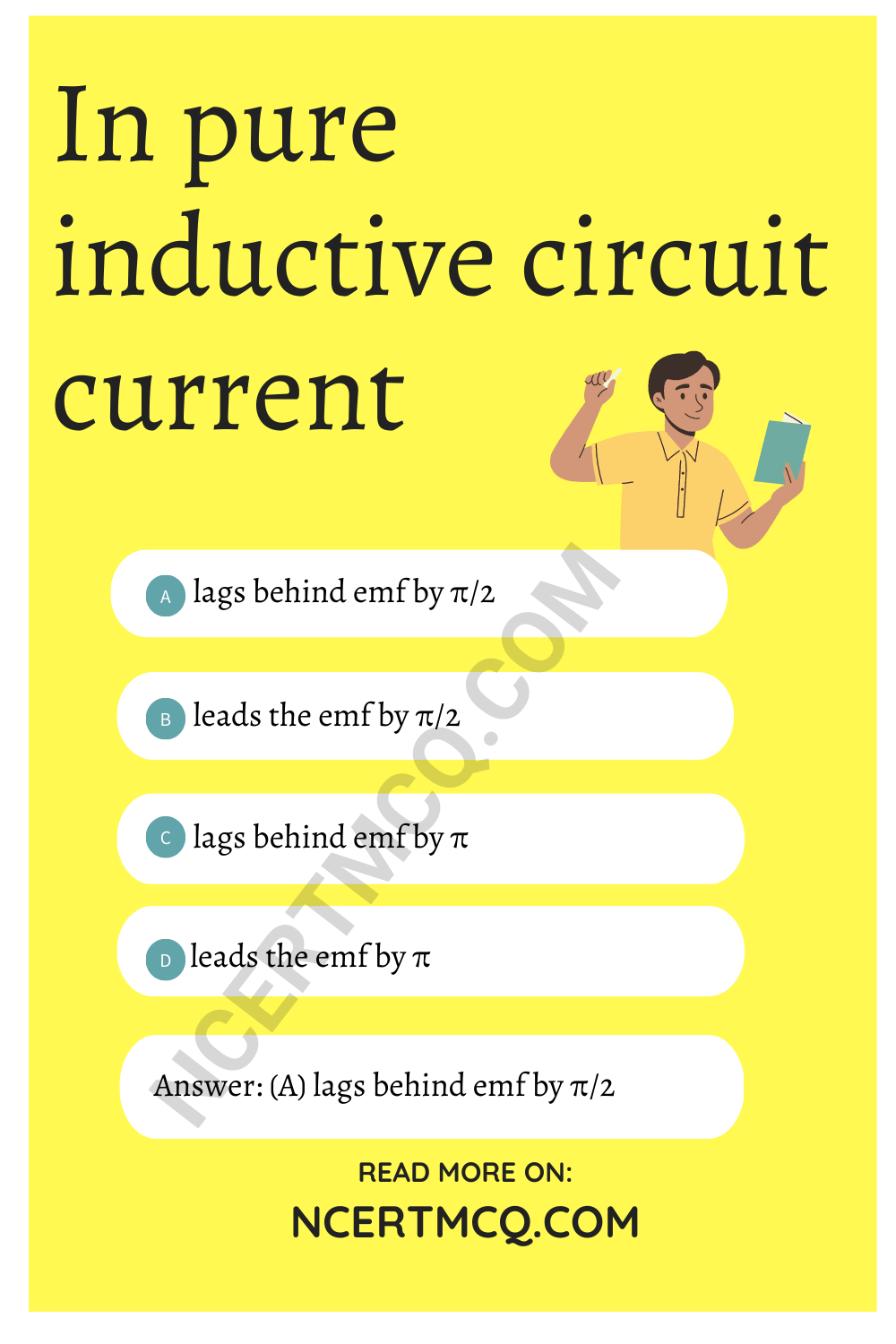Check the below NCERT MCQ Questions for Class 12 Physics Chapter 7 Alternating Current with Answers Pdf free download. MCQ Questions for Class 12 Physics with Answers were prepared based on the latest exam pattern. We have provided Alternating Current Class 12 Physics MCQs Questions with Answers to help students understand the concept very well.

## Alternating Current Class 12 MCQs Questions with Answers

Alternating Current Class 12 MCQ Question 1.
The average value of a cover a complete cycle is:
(a) l0
(b) $$\frac{l_0}{2}$$
(c) $$\frac{l_0}{√2}$$
(d) zero

Alternating Current MCQ Question 2.
Power factor of an ac circuit is a measure of:
(a) virtual power
(b) power lost in the circuit
(c) mean power
(d) all the above

Answer: (b) power lost in the circuit

Class 12 Physics Chapter 7 MCQ Question 3.
The dimensional formula of L/R is similar to that of:
(a) frequency
(b) time
(c) length
(d) none of these

Chapter 7 Physics Class 12 MCQ Question 4.
Which of the following has dimensions different from the rest?
(a) L/R
(b) $$\frac{1}{RC}$$
(c) $$\sqrt{LC}$$
(d) RC

Answer: (b) $$\frac{1}{RC}$$

MCQ On Alternating Current Question 5.
The dimensional formula of impedance is;
(a) [ML2T-2A-2]
(b) [ML2T-3A-2]
(c) [ML2T-2A-1]
(d) [ML2T-2A-3]

Class 12 Physics Chapter 7 MCQ Questions Question 6.
Energy dissipates in LCR circuit in :
(a) L only
(b) C only
(c) R only
(d) All of the aboveMCQ On Alternating Current Class 12 Question 7.
An iron core transformer with a turns ratio of 8 : 1 has 120 V applied across the primary. The voltage across the
(a) 15 V
(b) 120 V
(c) 180 V
(d) 960 V

MCQ Of Alternating Current Class 12 Question 8.
What is the ratio of inductive and capacitance reactances in an ac circuit?
(a) 1
(b) ω²L
(c) ω²LC
(d) l

Ch 7 Physics Class 12 MCQ Question 9.
An acceptor circuit is :
(a) series resonant circuit
(b) parallel resonant circuit
(c) LCR circuit
(d) None of these

Physics Class 12 Chapter 7 MCQ Question 10.
A broadcast circute broadcasts at 300 m band. A condenser of capacitance 2.4 lµF is available. The value of the inductance required for resonant circuit is:
(a) 10-4 H
(b) 10-8 H
(c) 10-6 H
(d) 10-2 H

Alternating Current MCQs Question 11.
In series resonant circuit:
(a) reactance is zero
(b) current is zero
(c) voltage is zero
(d) None of these

Class 12 Alternating Current MCQ Question 12.
In parallel resonant circuit:
(a) impedance is very high
(b) current is very high
(c) voltage is very high
(d) None of these

Answer: (a) impedance is very high

Class 12 Physics Ch 7 MCQ Question 13.
The no. of turns in the primary coil of a transformer is 200 and the no. of turns in the secondary is 10. If 240 V a.c. is applied to primary, the output from the secondary
(a) 6 V
(b) 12 V
(c) 24 V
(d) 48 V

Alternating Current Class 12 MCQ Questions Question 14.
Transformer works on the principle of:
(a) convertor
(b) invertor
(c) mutual induction
(d) self-induction

Alternating Current MCQ Class 12 Question 15.
Which of the following effects is not shown in alternating current ?
(a) Chemical effect
(b) Magnetic effect
(c) Heating current
(d) All of these

Physics Chapter 7 Class 12 MCQ Question 16.
An ac ammeter connected in series in an ac circuit reads 5A. The peak value of current:
(a) 5 A
(b) 5√2A
(c) $$\frac{5}{√5}$$ A
(d) zero

Alternating Current MCQ Questions And Answers Question 17.
The phase difference b/w the A.C. and e.m.f. $$\frac{π}{2}$$ Which of the following can not be the instituent of the circuit?
(a) LC
(b) L alone
(c) C alone
(d) RL

MCQ Questions For Class 12 Physics Chapter 7 Question 18.
The Q factor of an LCR circuit at resonance is :
(a) $$\sqrt{R^2+ω^2L^2}$$
(b) $$\sqrt{R^2+(\frac{1}{cω}})^2$$
(c) $$\frac{ω_rL}{R}$$
(d) $$\sqrt{R^2+\frac{1}{c^2ω^2}}$$

Answer: (c) $$\frac{ω_rL}{R}$$

Alternating Current Class 12 MCQs Question 19.
(a) depends on the amount of the electrolyte
(b) depends on the current in the electrolyte
(c) is a universal constant
(d) depends on the amount of charge passed through the electrolyte

Answer: (c) is a universal constant

Question 20.
The natural frequency of an L -C-Circuit is equal to :
(a) $$\frac{\sqrt{LC}}{2π}$$
(b) $$\frac{1}{3π\sqrt{LC}}$$
(c) $$\frac{1}{2π}\sqrt{\frac{L}{C}}$$
(d) $$\frac{1}{2π}\sqrt{\frac{C}{L}}$$

Answer: (b) $$\frac{1}{3π\sqrt{LC}}$$

Question 21.
In the series L-C-R circuit, the voltmeter and ammeter readings are:(a) V = 100 Volts, I = 2A
(b) V = 100 Volts, I = 5A
(c) V = 1000 Volts, I = 2A
(d) V = 300 Volts, I = 1A

Answer: (a) V = 100 Volts, I = 2A

Question 22.
The band width of a series resonant circuit is :
(a) f0/Q0
(b) $$\frac{R}{2πL}$$
(c) $$\frac{R}{4πL}$$
(d) 4R

Question 23.
A rejector circuit is:
(a) series resonant circuit
(b) parallel resonant circuit
(c) LCR circuit
(d) None of these

Question 24.
The inductive reactance of an inductor coil of $$\frac{1}{π}$$ H at 50 Hz is:
(a) $$\frac{50}{π}$$ Ω
(b) $$\frac{π}{50}$$ Ω
(c) 100 Ω
(d) 50 Ω

Question 25.
In pure inductive circuit current
(a) lags behind emf by π/2
(b) leads the emf by π/2
(c) lags behind emf by π
(d) leads the emf by π

Answer: (a) lags behind emf by π/2Question 26.
The current through an inductor of 1H is given by I = 3t sin t. The voltage across the inductor of 1 H is:
(a) 3 sin t + 3 cos t
(b) 3 sin t + 3t cos t
(c) 3 cos t + t sin t
(d) 3t cos t + sin t

Answer: (b) 3 sin t + 3t cos t

Question 27.
Two coils X and Y are connected in series across a 240 V, 50 Hz supply. The resistance of X is 5Ω and inductance of Y is 0.02 H. The power consumed is 3 kW and the power factor is 0.75. The impedance of the circuit is:
(a) 0.144 Ω
(b) 1.44 Ω
(c) 14.4 Ω
(d) 144 Ω

Question 28.
In the above problem, the value of the resistance of the coil Y is:
(a) 0.58 Ω
(b) 5.8 Ω
(c) 1.16 Ω
(d) 11.6 Ω

Question 29.
An electric circuit containing capacitor only suffers:
(a) equal resistance for A.C. and D.C.
(b) Resistance of A.C. > Resistance of D.C.
(c) Resistance for A.C. < Resistance of D.C.
(d) offers infinite resistance for D.C.

Answer: (d) offers infinite resistance for D.C.

Question 30.
The average power dissipation in pure inductance or capacitance is:
(a) $$\frac{1}{2}$$ LI²
(b) $$\frac{3}{2}$$ CV²
(c) either (a) or (b)
(d) zero